|

# 干货收藏:软装设计的十大经典美学原则

软装设计发源于欧洲，也被称为装饰派艺术。随着装饰、装修行业的发展和人们审美水平的不断提高，软装设计越来越多的走进了我们的生活，成为了装修设计中最为重要的一环。艺术，都是打造美的，也都有自身所对应的美学原则。

原则一：比例与技巧

原则要点：奥古斯丁曾经说过：'美是各部分的适当比例，再加一种悦目的颜色。'比例是物与物相对来说的，表明各种相对面间的度量关系，在美学中，最经典的比例分配莫过于'黄金分割'了；尺度是物与人（或其他易识别的不变要素）之间相比，不需涉及具体尺寸，完全凭感觉上的印象来把握。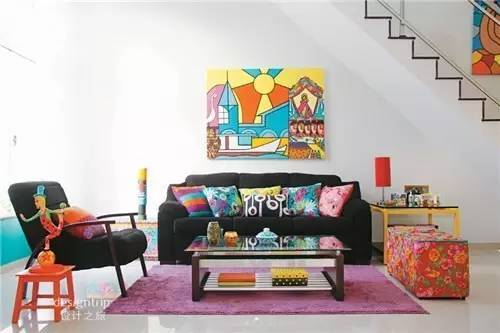应用技巧：比例是理性的、具体而标准的，可尺度却是感性的、抽象的。如果你没有特别的偏好，不妨就用1：0.618的比例来划居室空间吧，这是一个非常讨巧且有效的办法。原则二：稳定与轻巧

原则要点：稳定与轻巧几乎就是国人内心追求的写照，正统内敛、理性与感性兼容并蓄形成完美的生活方式。用这种心态来布置家居的话，与洛可可风格颇有不谋而合之处。以轻巧、自然、简洁、流畅为特点，将曲线运用发挥得淋漓尽致的洛可可式家具，在近年的复古风中极为时尚。应用技巧：稳定是整体，轻巧是局部。在居室内应用明快的色彩和纤巧的装饰，追求轻盈纤细的秀美。黄、绿、灰三色是客厅中的主要色彩。灰色向来给人稳重高雅的感觉，冲淡了灰的沉闷，而绿色中和了黄的耀眼，所有的布置都是为了最终形成稳定与轻巧的完美统一。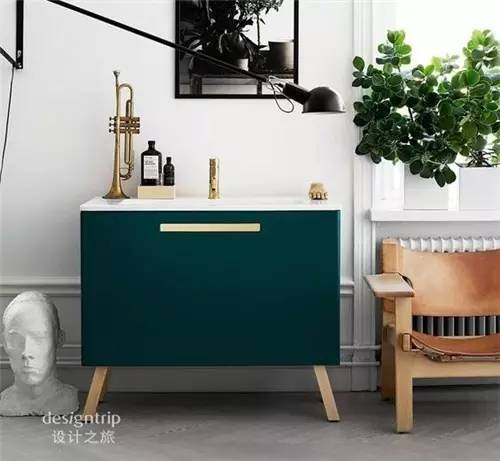注意事项：软装布置得过重会让人觉得压抑、沉闷；过轻又会让人觉得轻浮、毛躁。要注意色彩的轻重结合，家具饰物的形状大小分配协调，整体布局的合理完善等问题。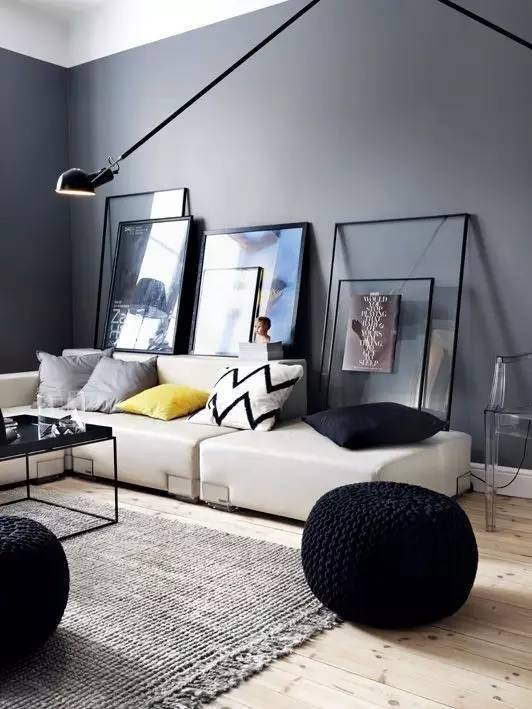原则三：调和与对比

原则要点：'对比'是美的构成形式之一，在家居布置中，对比手法的运用无处不在，可用做对比的角度也很多。比如通过光线的明暗对比、色彩的冷暖对比、材料的质地对比、传统与现代的对比等等。力求使家居风格产生更多层次、更多样式的变化，从而演绎出各种不同节奏的生活方式。而调和则是将对比双方进行缓冲与融合的一种有效手段。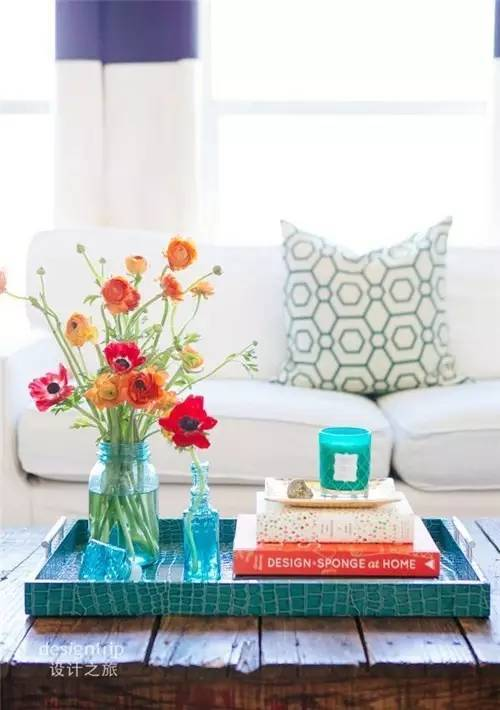注意事项：如果你有坚强的神经系统、独特的品味且我行我素、向来不惧人言，那么尽管使用强烈的对比吧，否则还是柔和一点的。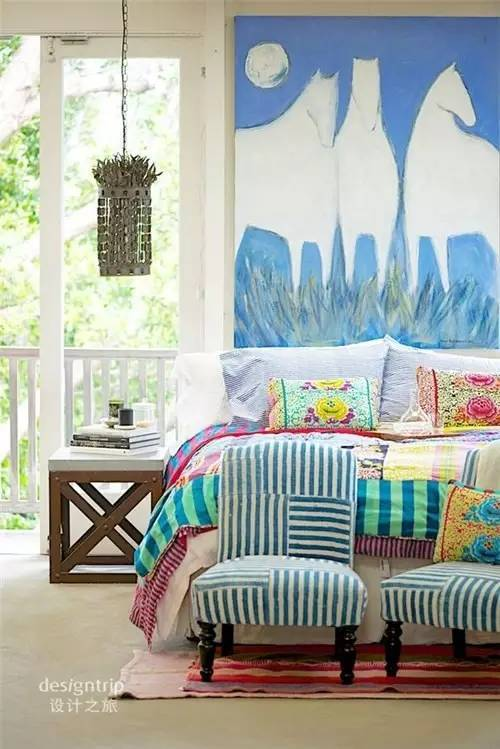原则四：节奏与韵律

原则要点：节奏与韵律是密不可分的统一体，是美感的共同语言，是创作和感受的关键。人称'建筑是凝固的音乐'，就是因为它们都是通过节奏与韵律的体现而造成美的感染力。成功的建筑总是以明确动人的节奏和韵律将无声的实体变为生动的语言和音乐，因而名扬于世。应用技巧：节奏与韵律是通过体量大小的区分、空间虚实的交替、构件排列的疏密、长短的变化、曲柔刚直的穿插等等变化来实现的，具体手法有：连续式、渐变式、起伏式、交错式等。楼梯是居室中最能体现节奏与韵律的所在。或盘旋而上、或蜿蜒起伏、或柔媚动人、或刚直不阿，每一部楼梯都可以做成一曲乐章，在家居中轻歌曼舞。注意事项：在整体软装中虽然可以采用不同的节奏和韵律，但同一个房间切忌使用两种以上的节奏，那会让人无所适从、心烦意乱。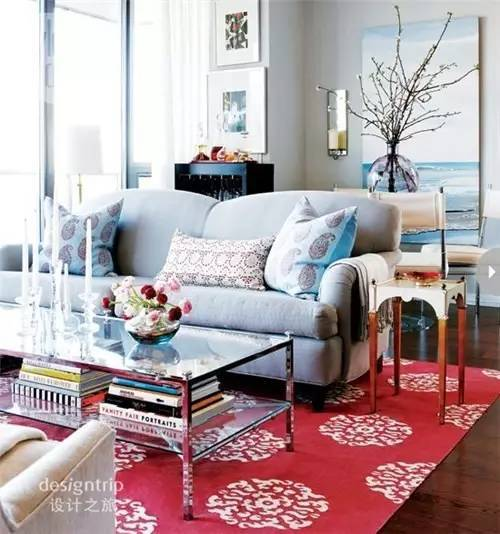原则五：对称与均衡

原则要点：对称是指以某一点为轴心，求得上下、左右的均衡。对称与均衡在一定程度上反映了处世哲学与中庸之道，因而在我国古典建筑中常常会运用到这种方式。现在居室装饰中人们往往在基本对称的基础上进行变化，造成局部不对称或对比，这也是一种审美原则。另有一种方法是打破对称，或缩小对称在室内装饰的应用范围，使之产生一种有变化的对称美。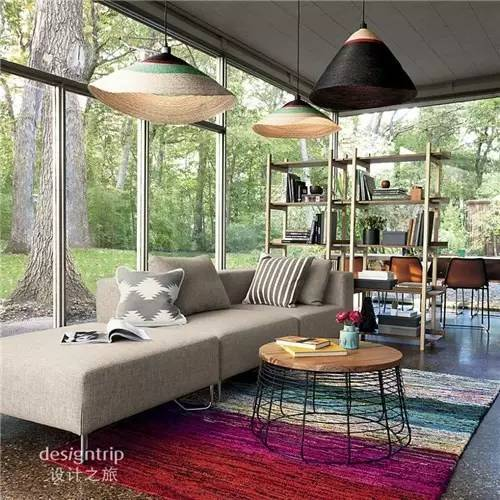应用技巧：面对庭院的落地大观景窗被匀称地划分成'格'，每一格中都是一幅风景。长方形的餐桌两边放着颜色相同，造型却截然不同的椅子、凳子，这是一种变化中的对称，在色彩和形式上达成视觉均衡。餐桌上的烛台和插花也是这种原则的体现。注意问题：对称性的处理能充分满足人的稳定感，同时也具有一定的图案美感，但要尽量避免让人产生平淡甚至呆板的感觉。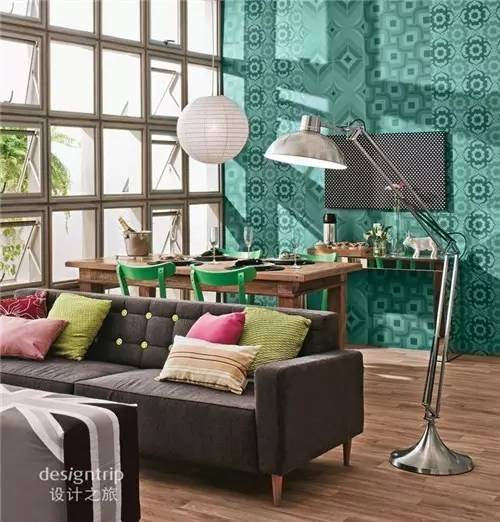原则六：主从与重点

原则要点：当主角和配角关系很明确时，心理也会安定下来。如果两者的关系模糊，便会令人无所适从，所以主从关系是软装布置中需要考虑的基本因素之一。在居室装饰中，视觉中心是极其重要的，人的注意范围一定要有一个中心点，这样才能造成主次分明的层次美感，这个视觉中心就是布置上的重点。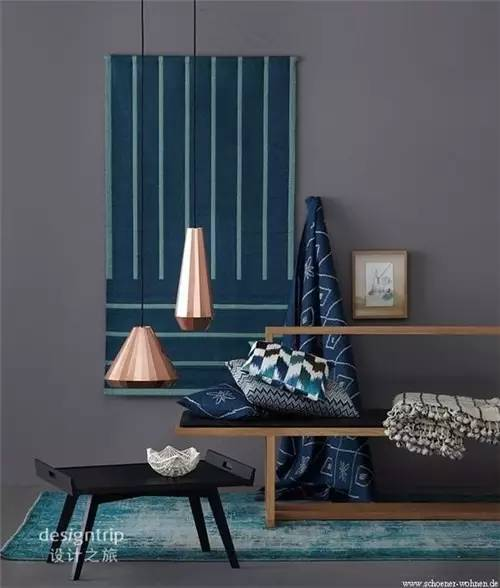注意事项：重点过多就会变成没有重点。配角的一切行为都是为了突出主角，切勿喧宾夺主。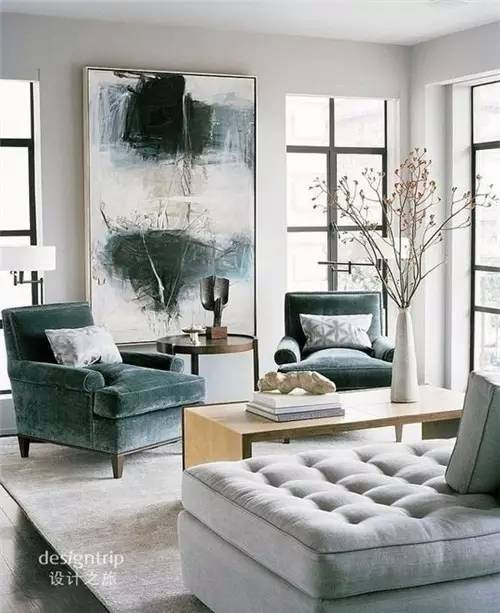原则七：过渡与呼应

原则要点：硬、软装修在色调、风格上的彼此和谐不难做到，难度在于如何让二者产生'联系'，这就需要运用'过渡'了。呼应属于均衡的形式美，是各种艺术常用的手法。在室内设计中，过渡与呼应总是形影相伴的，具体到顶棚与地面、桌面与墙面、各种家具之间......，形体与色彩层次过渡自然、巧妙呼应的话，往往能取得意想不到的效果。注意事项：'过渡与呼应'可以增加居室的丰富美感，但不宜太多或过分复杂，否则会给人造成杂乱无章及过于繁琐的感觉。原则八：比拟与联想

比拟是一种文学上的说法，在形式美学当中，它与联想密不可分。所谓联想，是指人们根据事物之间的某种联系由此及彼的心理思维过程。联想是联系眼前的事物与以往曾接触过的相似、相反或相关的事物之间的纽带和桥梁，它可以使人思路更开阔、视野更广远，从而引发审美情趣。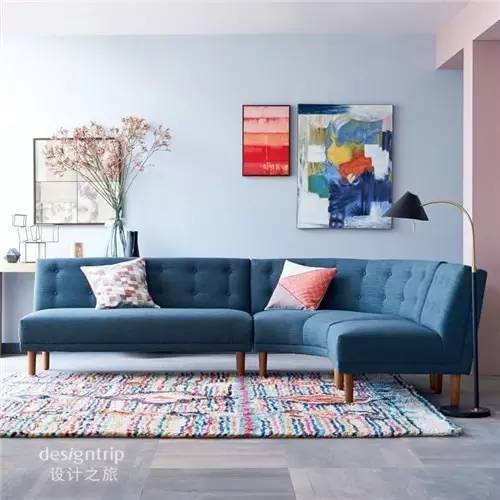联想的内容都是已知的、客观存在的，运用比拟手法，通过联想使抽象的意识活动与具体形象相结合。例如卧室，选用红调的布艺，再加上茂盛的绿色盆栽、立在窗边的长颈鹿摆饰，置身其中难免会从色彩、布景中产生热情洋溢、活力四射的非洲印象。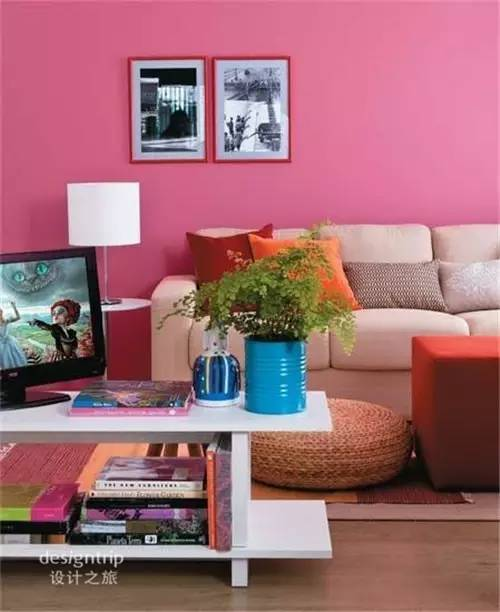运用这种原则布置家居时，一定要注意：比拟与联想从来都不是天马行空式的胡思乱想，它形成的空间应该是你曾经有过或者非常向往的生活氛围。原则九：同一与变化

软装布置在整体设计上应遵循'寓多样于统一'的形式美原则，根据大小、色彩、位置使之与家具构成一个整体，成为室内一景，营造出自然和谐、极具生命力的'统一与变化'；家具要有统一的艺术风格和整体韵味，最好成套定制或尽量挑选颜色、式样格调较为一致的，加上人文融合，进一步提升居住环境的品位。不同的空间应选用不同的色彩基调。黄色有助于人的食欲，所以将它定为餐厅的主色；墙上那幅青绿色的装饰画，是整体色调中的变数，然而却非常和谐；桌面、墙面、隔断采用相同花纹、相同材质，于统一见变化的是纹理方向的不同。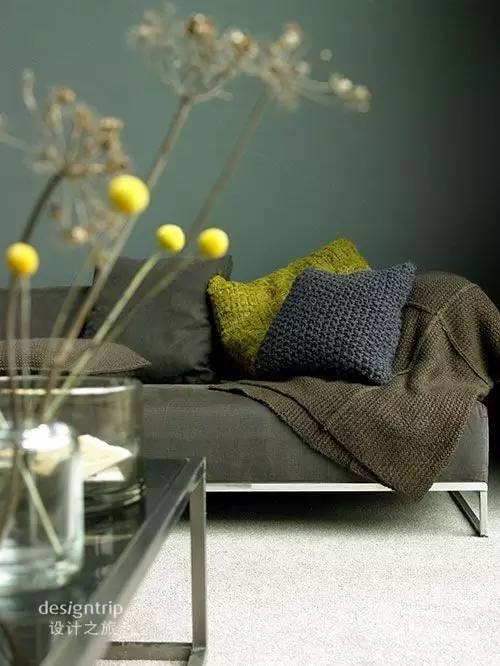在家居布置的初始就应该有一个完整的计划和构思，这样才不会在进行过程中出现纰漏；在购买新家具时，应尽量与原有家具般配。原则十：单纯风格

家居风格的成因是综合而复杂的，有意识形态的、有物质条件的、有传统的、有地域物产的，还有居住者个人的经历、才能及偏好和外来的影响等等因素。无论成因如何，首先要考虑好居室的基本风格，一旦建立起一种气氛，一种风格，一种角度，你就可以仔细地构建自己的风格，并且逐渐获得自信。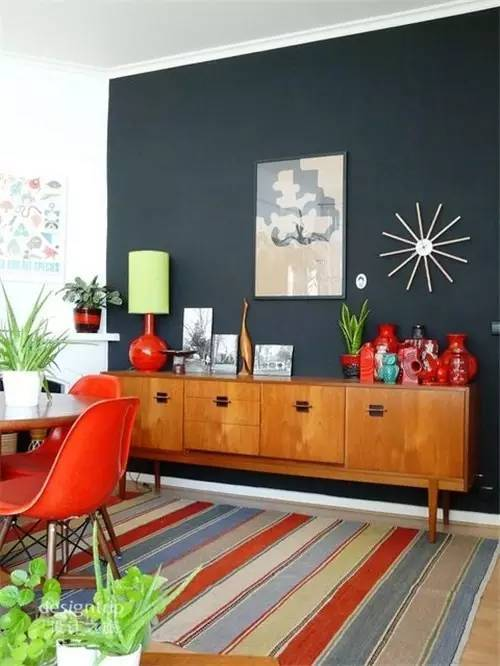人若单纯会让人感动，让人留恋。用在家居上，是一种返璞归真，一种洁净，一种清极而郁的芬芳。以原木为基调的卧室，素雅的布艺和生机盎然的绿色植物，不知不觉让人爱上它的纯净、它的境界、它的风平浪静。或许在人们的潜意识里，生活潮流总是希望有一种单纯的气质。`声明：本文由入驻焦点开放平台的作者撰写，除焦点官方账号外，观点仅代表作者本人，不代表焦点立场错误信息举报电话： 400-099-0099，邮箱：jubao@vip.sohu.com，或点此进行意见反馈，或点此进行举报投诉。`A B C D E F G H J K L M N P Q R S T W X Y Z
A - B - C - D - E
• A
• 鞍山
• 安庆
• 安阳
• 安顺
• 安康
• 澳门
• B
• 北京
• 保定
• 包头
• 巴彦淖尔
• 本溪
• 蚌埠
• 亳州
• 滨州
• 北海
• 百色
• 巴中
• 毕节
• 保山
• 宝鸡
• 白银
• 巴州
• C
• 承德
• 沧州
• 长治
• 赤峰
• 朝阳
• 长春
• 常州
• 滁州
• 池州
• 长沙
• 常德
• 郴州
• 潮州
• 崇左
• 重庆
• 成都
• 楚雄
• 昌都
• 慈溪
• 常熟
• D
• 大同
• 大连
• 丹东
• 大庆
• 东营
• 德州
• 东莞
• 德阳
• 达州
• 大理
• 德宏
• 定西
• 儋州
• 东平
• E
• 鄂尔多斯
• 鄂州
• 恩施
F - G - H - I - J
• F
• 抚顺
• 阜新
• 阜阳
• 福州
• 抚州
• 佛山
• 防城港
• G
• 赣州
• 广州
• 桂林
• 贵港
• 广元
• 广安
• 贵阳
• 固原
• H
• 邯郸
• 衡水
• 呼和浩特
• 呼伦贝尔
• 葫芦岛
• 哈尔滨
• 黑河
• 淮安
• 杭州
• 湖州
• 合肥
• 淮南
• 淮北
• 黄山
• 菏泽
• 鹤壁
• 黄石
• 黄冈
• 衡阳
• 怀化
• 惠州
• 河源
• 贺州
• 河池
• 海口
• 红河
• 汉中
• 海东
• 怀来
• I
• J
• 晋中
• 锦州
• 吉林
• 鸡西
• 佳木斯
• 嘉兴
• 金华
• 景德镇
• 九江
• 吉安
• 济南
• 济宁
• 焦作
• 荆门
• 荆州
• 江门
• 揭阳
• 金昌
• 酒泉
• 嘉峪关
K - L - M - N - P
• K
• 开封
• 昆明
• 昆山
• L
• 廊坊
• 临汾
• 辽阳
• 连云港
• 丽水
• 六安
• 龙岩
• 莱芜
• 临沂
• 聊城
• 洛阳
• 漯河
• 娄底
• 柳州
• 来宾
• 泸州
• 乐山
• 六盘水
• 丽江
• 临沧
• 拉萨
• 林芝
• 兰州
• 陇南
• M
• 牡丹江
• 马鞍山
• 茂名
• 梅州
• 绵阳
• 眉山
• N
• 南京
• 南通
• 宁波
• 南平
• 宁德
• 南昌
• 南阳
• 南宁
• 内江
• 南充
• P
• 盘锦
• 莆田
• 平顶山
• 濮阳
• 攀枝花
• 普洱
• 平凉
Q - R - S - T - W
• Q
• 秦皇岛
• 齐齐哈尔
• 衢州
• 泉州
• 青岛
• 清远
• 钦州
• 黔南
• 曲靖
• 庆阳
• R
• 日照
• 日喀则
• S
• 石家庄
• 沈阳
• 双鸭山
• 绥化
• 上海
• 苏州
• 宿迁
• 绍兴
• 宿州
• 三明
• 上饶
• 三门峡
• 商丘
• 十堰
• 随州
• 邵阳
• 韶关
• 深圳
• 汕头
• 汕尾
• 三亚
• 三沙
• 遂宁
• 山南
• 商洛
• 石嘴山
• T
• 天津
• 唐山
• 太原
• 通辽
• 铁岭
• 泰州
• 台州
• 铜陵
• 泰安
• 铜仁
• 铜川
• 天水
• 天门
• W
• 乌海
• 乌兰察布
• 无锡
• 温州
• 芜湖
• 潍坊
• 威海
• 武汉
• 梧州
• 渭南
• 武威
• 吴忠
• 乌鲁木齐
X - Y - Z
• X
• 邢台
• 徐州
• 宣城
• 厦门
• 新乡
• 许昌
• 信阳
• 襄阳
• 孝感
• 咸宁
• 湘潭
• 湘西
• 西双版纳
• 西安
• 咸阳
• 西宁
• 仙桃
• 西昌
• Y
• 运城
• 营口
• 盐城
• 扬州
• 鹰潭
• 宜春
• 烟台
• 宜昌
• 岳阳
• 益阳
• 永州
• 阳江
• 云浮
• 玉林
• 宜宾
• 雅安
• 玉溪
• 延安
• 榆林
• 银川
• Z
• 张家口
• 镇江
• 舟山
• 漳州
• 淄博
• 枣庄
• 郑州
• 周口
• 驻马店
• 株洲
• 张家界
• 珠海
• 湛江
• 肇庆
• 中山
• 自贡
• 资阳
• 遵义
• 昭通
• 张掖
• 中卫

1室1厅1厨1卫1阳台

1
2
3
4
5

0
1
2

1

1

0
1
2
3报名成功，资料已提交审核A B C D E F G H J K L M N P Q R S T W X Y Z
A - B - C - D - E
• A
• 鞍山
• 安庆
• 安阳
• 安顺
• 安康
• 澳门
• B
• 北京
• 保定
• 包头
• 巴彦淖尔
• 本溪
• 蚌埠
• 亳州
• 滨州
• 北海
• 百色
• 巴中
• 毕节
• 保山
• 宝鸡
• 白银
• 巴州
• C
• 承德
• 沧州
• 长治
• 赤峰
• 朝阳
• 长春
• 常州
• 滁州
• 池州
• 长沙
• 常德
• 郴州
• 潮州
• 崇左
• 重庆
• 成都
• 楚雄
• 昌都
• 慈溪
• 常熟
• D
• 大同
• 大连
• 丹东
• 大庆
• 东营
• 德州
• 东莞
• 德阳
• 达州
• 大理
• 德宏
• 定西
• 儋州
• 东平
• E
• 鄂尔多斯
• 鄂州
• 恩施
F - G - H - I - J
• F
• 抚顺
• 阜新
• 阜阳
• 福州
• 抚州
• 佛山
• 防城港
• G
• 赣州
• 广州
• 桂林
• 贵港
• 广元
• 广安
• 贵阳
• 固原
• H
• 邯郸
• 衡水
• 呼和浩特
• 呼伦贝尔
• 葫芦岛
• 哈尔滨
• 黑河
• 淮安
• 杭州
• 湖州
• 合肥
• 淮南
• 淮北
• 黄山
• 菏泽
• 鹤壁
• 黄石
• 黄冈
• 衡阳
• 怀化
• 惠州
• 河源
• 贺州
• 河池
• 海口
• 红河
• 汉中
• 海东
• 怀来
• I
• J
• 晋中
• 锦州
• 吉林
• 鸡西
• 佳木斯
• 嘉兴
• 金华
• 景德镇
• 九江
• 吉安
• 济南
• 济宁
• 焦作
• 荆门
• 荆州
• 江门
• 揭阳
• 金昌
• 酒泉
• 嘉峪关
K - L - M - N - P
• K
• 开封
• 昆明
• 昆山
• L
• 廊坊
• 临汾
• 辽阳
• 连云港
• 丽水
• 六安
• 龙岩
• 莱芜
• 临沂
• 聊城
• 洛阳
• 漯河
• 娄底
• 柳州
• 来宾
• 泸州
• 乐山
• 六盘水
• 丽江
• 临沧
• 拉萨
• 林芝
• 兰州
• 陇南
• M
• 牡丹江
• 马鞍山
• 茂名
• 梅州
• 绵阳
• 眉山
• N
• 南京
• 南通
• 宁波
• 南平
• 宁德
• 南昌
• 南阳
• 南宁
• 内江
• 南充
• P
• 盘锦
• 莆田
• 平顶山
• 濮阳
• 攀枝花
• 普洱
• 平凉
Q - R - S - T - W
• Q
• 秦皇岛
• 齐齐哈尔
• 衢州
• 泉州
• 青岛
• 清远
• 钦州
• 黔南
• 曲靖
• 庆阳
• R
• 日照
• 日喀则
• S
• 石家庄
• 沈阳
• 双鸭山
• 绥化
• 上海
• 苏州
• 宿迁
• 绍兴
• 宿州
• 三明
• 上饶
• 三门峡
• 商丘
• 十堰
• 随州
• 邵阳
• 韶关
• 深圳
• 汕头
• 汕尾
• 三亚
• 三沙
• 遂宁
• 山南
• 商洛
• 石嘴山
• T
• 天津
• 唐山
• 太原
• 通辽
• 铁岭
• 泰州
• 台州
• 铜陵
• 泰安
• 铜仁
• 铜川
• 天水
• 天门
• W
• 乌海
• 乌兰察布
• 无锡
• 温州
• 芜湖
• 潍坊
• 威海
• 武汉
• 梧州
• 渭南
• 武威
• 吴忠
• 乌鲁木齐
X - Y - Z
• X
• 邢台
• 徐州
• 宣城
• 厦门
• 新乡
• 许昌
• 信阳
• 襄阳
• 孝感
• 咸宁
• 湘潭
• 湘西
• 西双版纳
• 西安
• 咸阳
• 西宁
• 仙桃
• 西昌
• Y
• 运城
• 营口
• 盐城
• 扬州
• 鹰潭
• 宜春
• 烟台
• 宜昌
• 岳阳
• 益阳
• 永州
• 阳江
• 云浮
• 玉林
• 宜宾
• 雅安
• 玉溪
• 延安
• 榆林
• 银川
• Z
• 张家口
• 镇江
• 舟山
• 漳州
• 淄博
• 枣庄
• 郑州
• 周口
• 驻马店
• 株洲
• 张家界
• 珠海
• 湛江
• 肇庆
• 中山
• 自贡
• 资阳
• 遵义
• 昭通
• 张掖
• 中卫• 手机• 分享
• 设计
免费设计
• 计算器
装修计算器
• 入驻
合作入驻
• 联系
联系我们
• 置顶
返回顶部